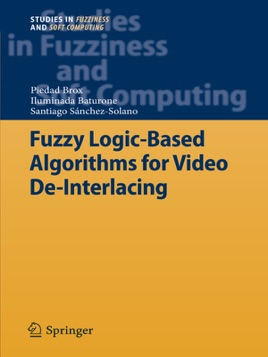• 119,99 €

## Description de l’éditeur

The ‘Fuzzy Logic’ research group of the Microelectronics Institute of Seville is composed of researchers who have been doing research on fuzzy logic since the beginning of the 1990s. Mainly, this research has been focused on the microelectronic design of fuzzy logic-based systems using implementation techniques which range from ASICs to FPGAs and DSPs. Another active line was the development of a CAD environment, named Xfuzzy, to ease such design. Several versions of Xfuzzy have been and are being currently developed by the group. The addressed applications had basically belonged to the control field domain. In this sense, several problems without a linear control solution had been studied thoroughly. Some examples are the navigation control of an autonomous mobile robot and the level control of a dosage system.

This book is organized in five chapters. In Chapter 1, some basic concepts are explained to completely understand the contribution of the algorithms developed in this research work. The evaluation of how motion is present and how it influences on de-interlacing is studied in Chapter 2. The design options of the proposed fuzzy motion-adaptive de-interlacing algorithm is studied in Chapter 3. A spatial interpolator that adapts the interpolation to the presence of edges in a fuzzy way is developed in Chapter 4. A temporal interpolator that adapts the strategy of the interpolation to possible repetition of areas of fields is presented in Chapter 5. Using both interpolators in the fuzzy motion-adaptive algorithm described in Chapter 3 clearly improves the de-interlaced results.